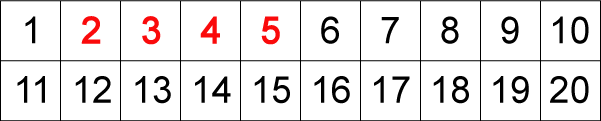#### You may also like### Month Mania

Can you design a new shape for the twenty-eight squares and arrange the numbers in a logical way? What patterns do you notice?### Starfish Spotting

How many starfish could there be on the beach, and how many children, if I can see 28 arms?### Catrina's Cards

How would you find out how many football cards Catrina has collected?

# What's Left?

##### Age 5 to 7Challenge Level

Here is a grid showing the numbers $1-20$:Start with the first red number, $2$. Now, look along the top row of the grid to the next number, $3$.

Can you share $3$ exactly between $2$?

If the answer is yes then shade in the $3$ box. If the answer is no, leave it.

Now take the next number, $4$. Can you share $4$ exactly between $2$?

Again, if you can, shade the box with $4$ in it. Carry on doing the same with all the numbers.

Now do the same with the next red number, $3$. Look along the row for the next number, $4$ and shade all the numbers that will share exactly between $3$.

Now try with $4$ and with $5$.

Which numbers do you have left?